## From the top of a cliff, a person throws a stone straight downward. The initial speed of the stone just after leaving the person’s hand is 9

Question

From the top of a cliff, a person throws a stone straight downward. The initial speed of the stone just after leaving the person’s hand is 9.7 m/s. (a) What is the acceleration (magnitude and direction) of the stone while it moves downward, after leaving the person’s hand? magnitude m/s^2direction Is the stone’s speed increasing or decreasing? a. increasing b. decreasingAfter 0.51 s, how far beneath the top of the cliff is the stone? (Give just the distance fallen, that is, a magnitude.)________ m.

in progress 0
6 months 2021-08-02T15:36:39+00:00 1 Answers 19 views 0

a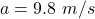Increasing

b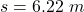Explanation:

From the question we are told that

The  initial speed is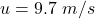The  time  taken is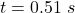Generally given that the stone is moving downward the acceleration is  equivalent to the generally value of acceleration due to gravity and it would be increasing as the stone approaches the ground(toward the center of the earth )

Thus the acceleration isGenerally from the equation of motion we have that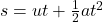=>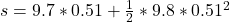=>